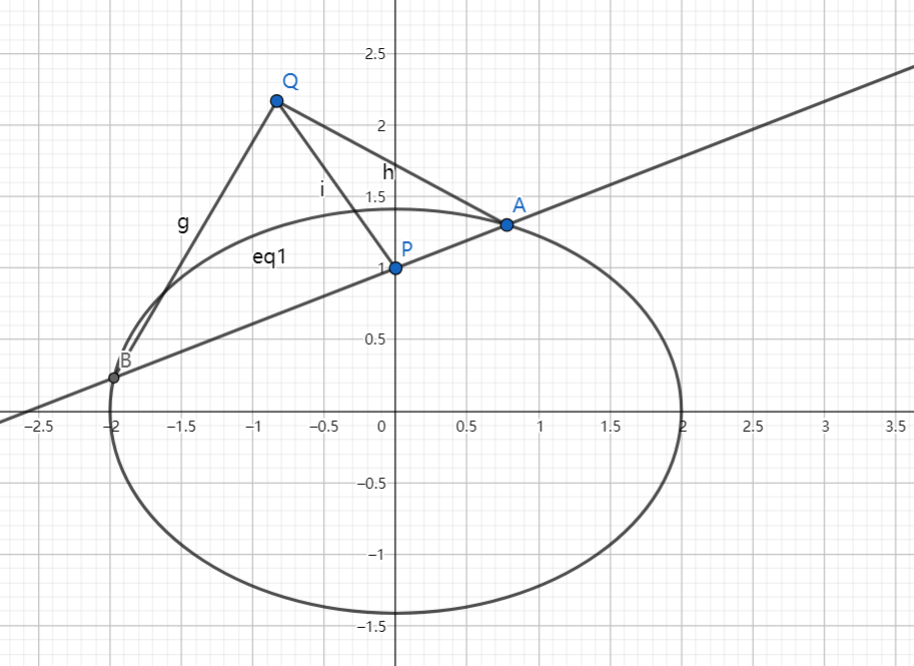# SAT1000 - P842

Geometry Level pendingAs shown above, the ellipse has equation: $\dfrac{x^2}{4}+\dfrac{y^2}{2}=1$, and line $l$ passing through $P(0,1)$ intersects with the ellipse at point $A,B$.

Then there exists a fixed point $Q$ so that the following equation always holds as $l$ rotates:

$\dfrac{|QA|}{|QB|}=\dfrac{|PA|}{|PB|}$

Then find the coordinate of $Q$.

The coordinate of $Q$ is $(x_0,y_0)$. Submit $\lfloor 1000(2y_0-x_0) \rfloor$.

Have a look at my problem set: SAT 1000 problems

×